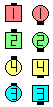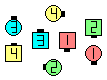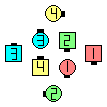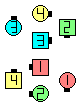Definitions of Square Dance Calls and Concepts
Wrap To A {formation} [C3A]

Index -->  Plus  |  A1  |  A2  |  C1  |  C2  |  C3A  |  C3B  |  C4  |  NOL  |
Definitions (Text Only) -->  Plus  |  A1  |  A2  |  C1  |  C2  |  C3A  |  C3B  |  C4  |  NOL  |
 Find call:

Wrap To A formation -- [C3A]
(Lee Kopman 1976)
 C3A:\$B8@8l(BColumn \$B\$+\$i!%(B

1 \$BHVL\\$N?M(B:
1/2 Split Circulate \$B\$H(B Phantom formation Circulate 2.
2 \$BHVL\\$N?M(B:
Circulate, 1/2 Split Circulate, \$B\$H(B Phantom formation Circulate.
3 \$BHVL\\$N?M(B:
Circulate 2 & 1/2.
4 \$BHVL\\$N?M(B:
Circulate 3.

\$B=*\$o\$j\$GI,MW\$G\$"\$l\$P(B, \$BM?\$(\$i\$l\$?(B formation \$B\$K(B Adjust \$B\$7\$^\$9!%(B\$B\$B;O\$a\$N(B formation:Wrap To An Hourglass\$B\$N8e(BWrap To A Galaxy\$B\$N8e(BWrap To Interlocked Diamonds\$B\$N8e(B

\$BCm
• \$BA40w\$,9g7W(B 3 \$B\$D\$N(B Circulate (\$B\$^\$?\$O(B Circulate \$B\$NItJ,(B) \$B\$r\$7\$^\$9!%(B
• 1 \$BHVL\\$N?M\$O(B, \$B>o\$K7k2L\$N(B formation \$B\$N(B Very Center \$B\$+(B Center Line \$B>e\$G=*\$o\$j\$^\$9!%(B
• formation \$B\$K\$O(B, (Twin) Diamonds \$B\$d(B Point-to-Point Diamonds \$B\$b\$"\$j\$^\$9!%(B
• Wrap To A formation \$B\$O(B Unwrap The formation \$B\$N(B Rewind \$B\$G\$9!%(B \$B\$9\$J\$o\$A(B, "Wrap To A formation; U-Turn Back; Unwrap The formation; U-Turn Back" \$B\$N(B sequence \$B\$O(B zero \$B\$G\$9!%(B \$BLu
•Choreography for Wrap To A formationComments? Questions? Suggestions?

 C3A:https://www.ceder.net/def/wrap.php?language=japan&level=C3A
21-January-2020 06:05:33